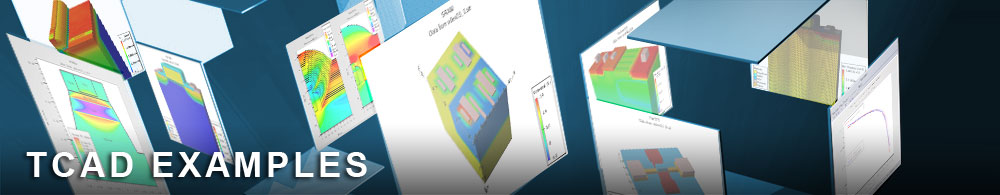Sub-Threshold Slope Extraction

## mos1ex10.in : Sub-Threshold Slope Extraction

Requires: SSuprem 4/S-Pisces
Minimum Versions: Athena 5.22.3.R, Atlas 5.28.1.R

This is a basic MOS Athena to Atlas interface example simulating an Id/Vgs curve and extracting sub-threshold slope. No advanced features are used in this example so as to demonstrate simple functionality. This example demonstrates:

• Process simulation of a MOS transistor in Athena
• Process parameter extraction (eg. oxide thicknesses)
• Autointerface between Athena and Atlas
• Simple Id/Vgs curve generation with Vds=-0.1V
• Parameter extraction for Sub-Threshold Slope

The process simulation, process parameter extraction and electrode definition for this example are exactly as described in the first example in this section.

In Atlas the same sequence of statements is used as in the first example in this section. The only difference is that the gate voltage is ramped from zero to -1.0V only in -0.1V steps.

The extract statement used in this example measures the sub-threshold slope of the MOSFET. The syntax uses the operators log10(), slope() and curve() to specify the reciprocal of the steepest slope to the curve of Vgs vs. log(Id). Note that the operator log10() is needed here. The operator log() specifies the function for the natural logarithm. It is obviously necessary to take the absolute value of Id before the log10() operator is used.

To load and run this example, select the Load button in DeckBuild > Examples. This will copy the input file and any support files to your current working directory. Select the Run button in DeckBuild to execute the example.